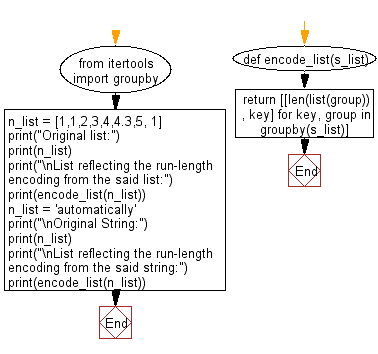﻿ Python: Create a list reflecting the modified run-length encoding - w3resource# Python: Create a list reflecting the modified run-length encoding

## Python List: Exercise - 76 with Solution

Write a Python program to create a list reflecting the modified run-length encoding from a given list of integers or a given list of characters.

Sample Solution:

Python Code:

``````from itertools import groupby
def modified_encode(alist):
def ctr_ele(el):
if len(el)>1: return [len(el), el]
else: return el
return [ctr_ele(list(group)) for key, group in groupby(alist)]

n_list = [1,1,2,3,4,4,5, 1]
print("Original list:")
print(n_list)
print("\nList reflecting the modified run-length encoding from the said list:")
print(modified_encode(n_list))

print("\nOriginal String:")
print(n_list)
print("\nList reflecting the modified run-length encoding from the said string:")
print(modified_encode(n_list))

```
```

Sample Output:

```Original list:
[1, 1, 2, 3, 4, 4, 5, 1]
List reflecting the modified run-length encoding from the said list:
[[2, 1], 2, 3, [2, 4], 5, 1]

Original String:

List reflecting the modified run-length encoding from the said string:
[[2, 'a'], 'b', 'c', [4, 'd'], 'a', 'd', 'n', [2, 's']]
```

Flowchart:## Visualize Python code execution:

The following tool visualize what the computer is doing step-by-step as it executes the said program:

Python Code Editor:

Have another way to solve this solution? Contribute your code (and comments) through Disqus.

What is the difficulty level of this exercise?

Test your Python skills with w3resource's quiz

﻿

## Python: Tips of the Day

```print(2_000_000)
```2000000